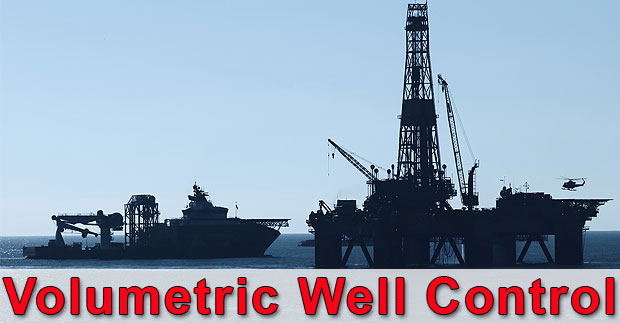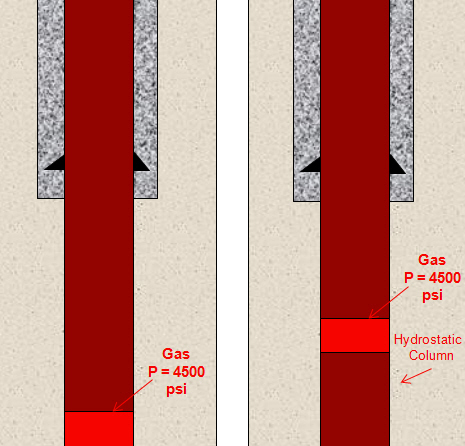# Volumetric Well Control – When It Will Be Used

Volumetric well control method is a special well control method which will be used when the normal circulation cannot be done. It is not a kill method but it the method to control bottom hole pressure and allow influx to migrate without causing any damage to the well.There are several situations where you cannot circulate the well as follows:

• Pumps broken down

• Plugged drill string/bit

• Drill string above the kick

• Drill string is out of the hole completely

With the volumetric method, the volume of gas influx will allow migrating and casing pressure will increase till a certain figure then a specific amount of mud will bleed off to compensate the increase in casing pressure. The volumetric method will allow the kick to surface while the bottom hole pressure is almost constant. Successful use of volumetric method requires personnel understand three basic concepts –

1. Boyle’s Law – Boyle’s law states that at constant temperature, the absolute pressure and the volume of a gas are inversely proportional in case of constant temperature within a closed system. The illustration below demonstrates volume and pressure as per Boyle’s Law.In term of mathematical relationship, Boyle’s Law can be stated as

P1 x V1 = P2 x V1

Where;

P1 = pressure of gas at the first condition

V1 = volume of gas at the first condition

P2 = pressure of gas at the second condition

V2 = volume of gas at the second condition

2. Hydrostatic pressure – Hydrostatic pressure is pressure created by column of fluid. Two factors affecting hydrostatic pressure are height of fluid and density of fluid.

Pressure at the bottom hole equals to hydrostatic pressure plus surface pressure

Pressure (bottom hole) = Hydrostatic Pressure + Surface Pressure

We will apply this concept to see how the gas bubble will increase the bottom hole pressure.

If the gas bubble is not allowed to expanded, the gas bubble in the well migrates up will act on the mud column below and increase bottom hole pressure. Increasing in the bottom hole pressure equates to hydrostatic pressure below the bubble.

Bottom hole pressure = Gas bubble pressure + Hydrostatic pressure below the bubbleIf we don’t want increase in bottom hole pressure, mud need to be bled off the well while the gas migrating up and the casing pressure must increase to compensate loss of hydrostatic pressure from bleed off.

In the volumetric control, there are two ways to control bottom hole pressure while allowing the gas migrating up to surface.

1. Wait and let gas migrate. The migration of gas will increase bottom hole pressure and casing pressure.

2. Bleed off mud from the annulus. Mud that is bled off must be equal to the increase in bottom hole pressure.

Both steps above must be carefully performed perform in a sequence. We will go to the detailed procedures in later post.

3. Relationship of height and fluid volume as determined by annular capacity – In order to determine volume of mud that equates to required hydrostatic pressure, we need to understand annulus capacity. It tells us how many bbl per foot in annulus and it can be calculated by this following formulas:

Annular Capacity Factor (ACF) = (OD2-ID2) ÷ 1029.4

Where;

ACF = Annular Capacity Factor in bbl/ft

OD = Outside Diameter of Annular in inch

ID = Inside Diameter of Annular in inch

Once the ACF is know, we can determine Mud Increment (MI) which is the volume of mud bled off from the annulus to reduce the annular hydrostatic pressure by the amount of the pressure required.

Mud Increment (MI) can be calculated by this following equation:

Mud Increment (MI) = (PI x ACF) ÷ (0.052 x MW)

Where;

PI = Pressure Increment in psi

ACF = Annular Capacity Factor in bbl/ft

MW = Mud Weight in the well in ppg

More details about volumetric well control can be found in the following articles;

References

Cormack, D. (2007). An introduction to well control calculations for drilling operations. 1st ed. Texas: Springer.

Crumpton, H. (2010). Well Control for Completions and Interventions. 1st ed. Texas: Gulf Publishing.

Grace, R. (2003). Blowout and well control handbook [recurso electrónico]. 1st ed. Paises Bajos: Gulf Professional Pub.

Grace, R. and Cudd, B. (1994). Advanced blowout & well control. 1st ed. Houston: Gulf Publishing Company.

Watson, D., Brittenham, T. and Moore, P. (2003). Advanced well control. 1st ed. Richardson, Tex.: Society of Petroleum Engineers.Working in the oil field and loving to share knowledge.

### 3 Responses to Volumetric Well Control – When It Will Be Used

1.Artelgash says:

The MI (bbls) calculation is premised on a vertical well, for directional well (especially Hi-Angle), do we need to consider a factor Cos (a) of MI (bbls). For example, if the hole angle is 60 degrees, the factor Cos(60。) = 0.5, which means MI (bbls) should be two times greater than that of vertical well. MI = (PIxACF)/(0.052xMWxCos (a)), I know it’s difficult to be precise. Or this could give us a better idea on how to control mud bleed-off effectively and safely. Thanks in advance for your comments on this.

2.Fregene Godwin says:

3.Riyadh says: